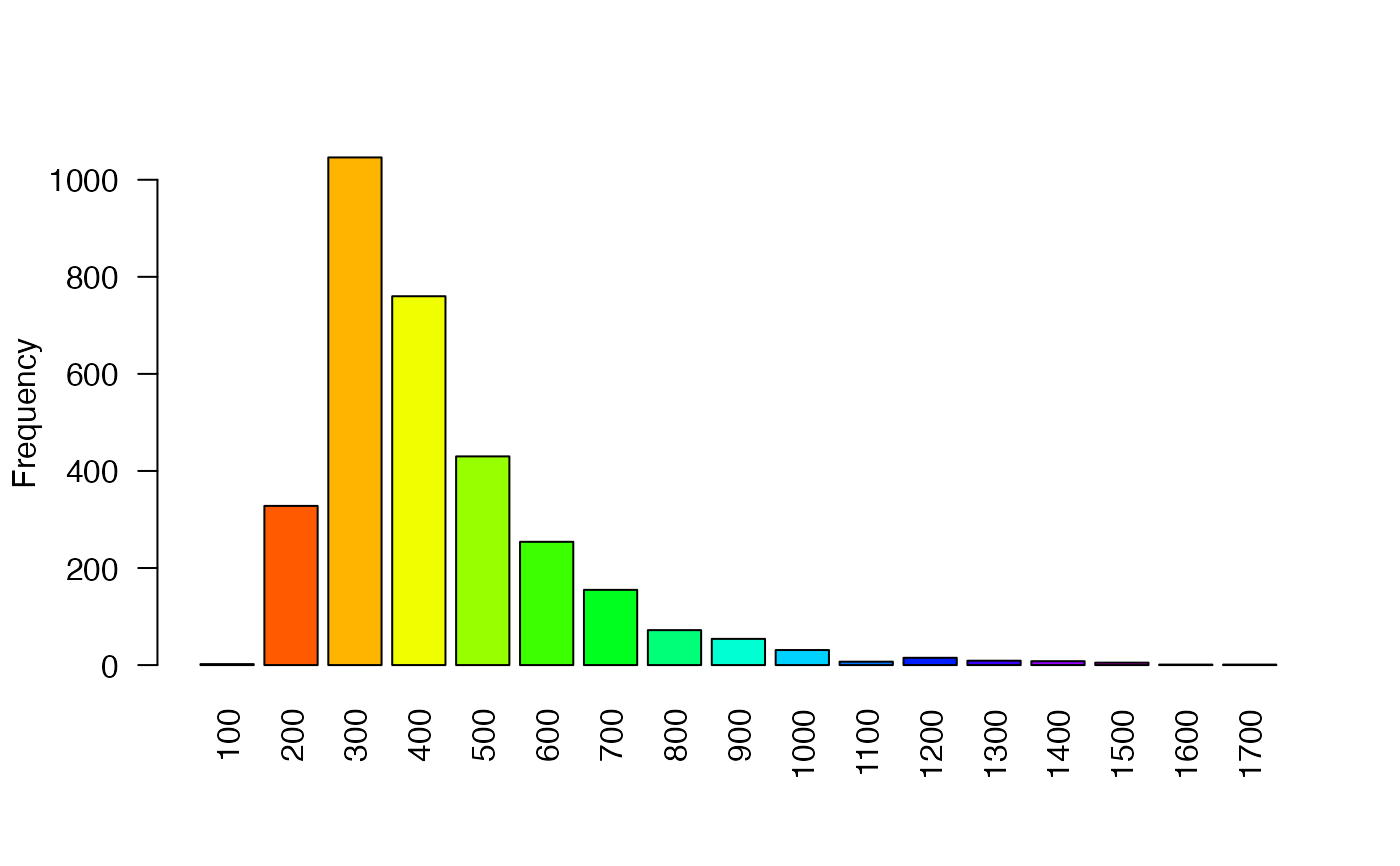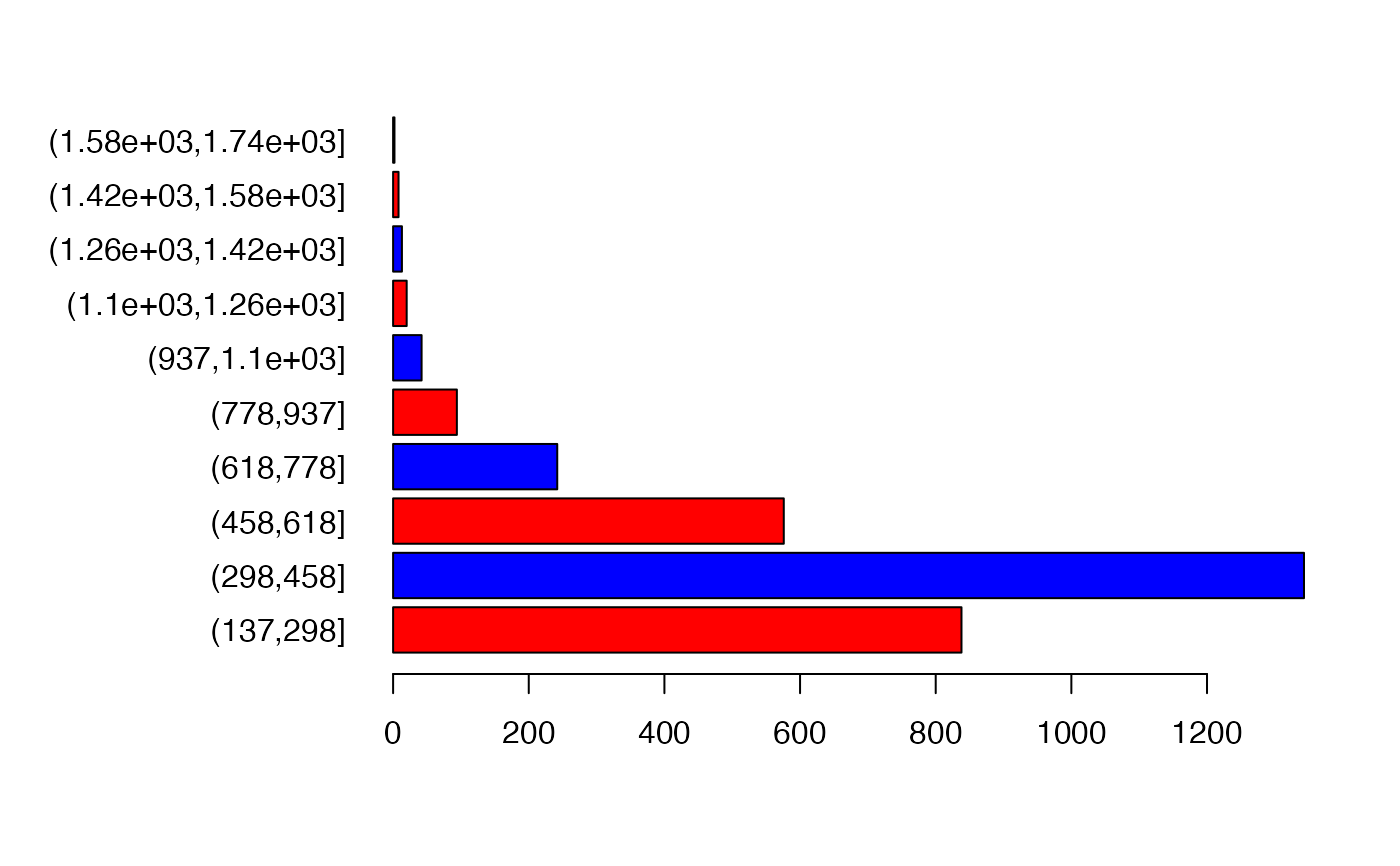Create a barplot of the values of a RasterLayer. For large datasets a regular sample with a size of approximately maxpixels is used.

# S4 method for RasterLayer
barplot(height, maxpixels=1000000, digits=0, breaks=NULL, col=rainbow, ...)

## Arguments

height

RasterLayer

maxpixels

integer. To regularly subsample very large objects

digits

integer used to determine how to round the values before tabulating. Set to NULL or to a large number if you do not want any rounding

breaks

breaks used to group the data as in cut

col

a color generating function such as rainbow, or a vector of colors

...

additional arguments for plotting as in barplot

hist, boxplot

## Value

A numeric vector (or matrix, when beside = TRUE) of the coordinates of the bar midpoints, useful for adding to the graph. See barplot

## Examples

f <- system.file("external/test.grd", package="raster")
r <- raster(f)
barplot(r, digits=-2, las=2, ylab='Frequency')